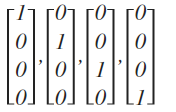# 机器学习3

## 神经网络

### 神经元和大脑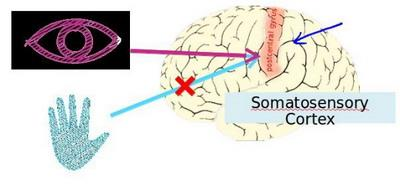### 模型展示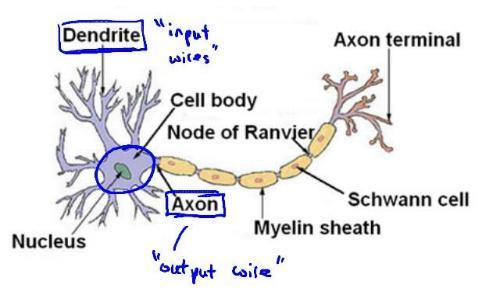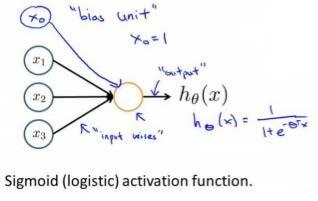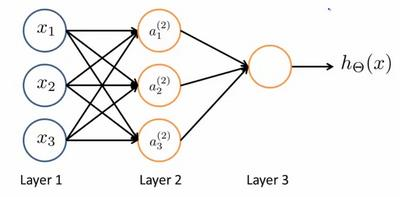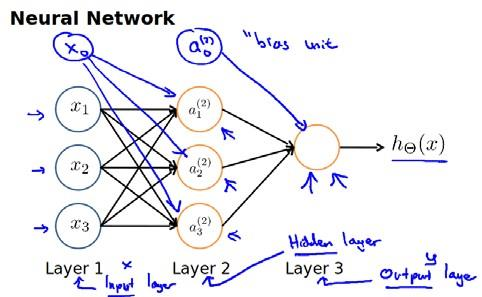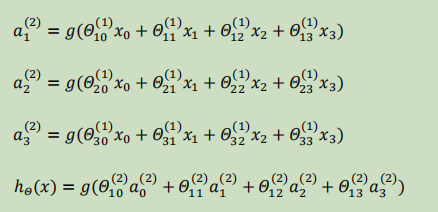$\theta^{(j)}$为$s_{j+1}\times(s_j+1)$维度，即第j+1层单元数乘以第j层单元数+1【偏差单元】的和

### 例子与直观理解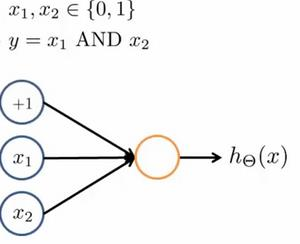eg：θ0=-30, θ1=20, θ2=20

hθ(x) = g(−30 + 20x1 +20x2)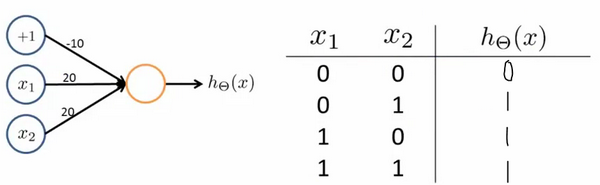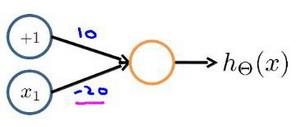XNOR = (x1 AND x2) OR((NOT x1)AND(NOT x2))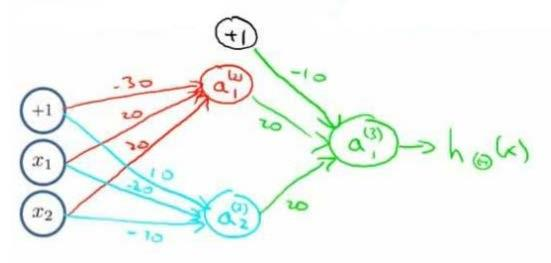### 多元分类

eg：每个向量中为1的为一类